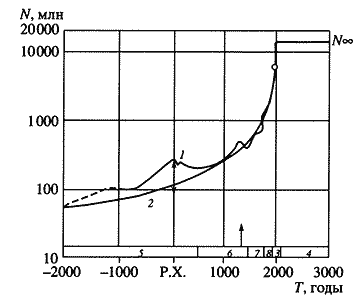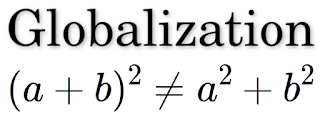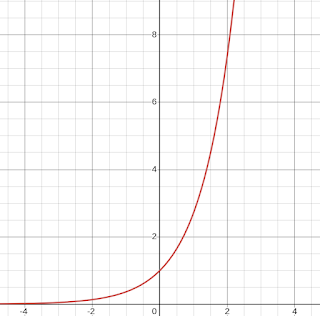## Posts

### Innovation vs. Creativity (or Tactics vs. Strategy)

Nokia Nokia. My first cellular phone was Nokia. Those days Nokia created best cellular phones. Nokia, once a dominant force in the mobile phone industry, has experienced a dramatic decline. From a market capitalization of $550 billion in 2000, it plummeted to just$18 billion. This decline prompts a critical question: Why did Nokia fail while companies like Apple soared to unprecedented heights ? While Nokia excelled at producing high-quality cellular phones, it missed the boat on the smartphone revolution. Smart phones slowly gain more and more customers, and, of course, who bought smart phone did not buy cellular phone. Nokia did not lost it's domain of cellular phones. Nokia just missed this moment when new market of smartphones was created. Those two markets could not coexist. Such situation defined by " Struggle of Informations " model as "antagonistic informations" where only one information will survive and other will totally disappear. The critical ques

### 2025 - Global Demographic TransitionWe stand at a pivotal moment in human history, not merely facing another crisis but confronting a fundamental shift in our existence. This is not a passing storm but a transformation that demands our attention and understanding. 2025 In my previous blog post " Globalization "  I delved into the demographic research of Russian professor Sergey Kapitsa, " PARADOXES OF GROWTH: LAWS OF GLOBAL DEVELOPMENT OF HUMANITY ".  Kapitsa identified a mathematical model that accurately represents global human population growth, expressed by the differential equation: \begin{equation} \large{}\color{blue}\frac{d N}{d T} = \frac{N^2}{2*10^{11}} \end{equation}Professor Kapitsa presented human population growth not only in form of differential eqation. He also introduced formal formula for calculating human population over time.\begin{equation} \large{}\color{blue}N = \frac{2*10^{11}}{2025-T} \end{equation}where N is number of people over globe and T is year. In picture you can s

### GlobalizationIn my previous post, " Life, Death, and 'The Winner Takes It All' " I discussed a simple differential equation that can be used to calculate population size \begin{equation} \large{}\color{blue}\frac{d x}{d t} = x \end{equation} This equation, known as the exponential function, becomes more complex when it incorporates death rates (the logistic function) and competition (the "struggle of information" model). However, the underlying principle of these models remains the same: the growth rate of a population is proportional to its current size. This principle holds true for most species, but there is one notable exception: humans. Humans are unique in that their population growth does not follow the basic principle outlined above. The study of human population size and growth rates is known as demography. Demographers collect statistics on the number of people in different areas and develop mathematical models to represent these numbers as accura

### "Life", "Death" and "The Winner Takes It All"I want to talk about how one simple differential equation became very useful mathematical model that reflect how different ecosystems (both in nature and in technology) evolve. "Life" One of most known ecosystems used for mathematical modeling is population of rabbits. We assume that next generation of rabbits will have all already living rabbits plus new born rabbits. Number of new born rabbits is proportional to number of existing rabbits. We'll take simplest case when number of additional rabbits equal to number of existing rabbits (when 2 rabbits bring 2 new rabbits). This means that change of number of rabbits equal to number of rabbits, or as differential equation: \begin{equation} \large{}\color{blue}\frac{d x}{d t} = x \end{equation} General solution for this differential equation is: \begin{equation} \Large{}\color{blue} x = e^t \end{equation}(where X is population number, T is time). This equation is known as " exponential function ". Many times thi